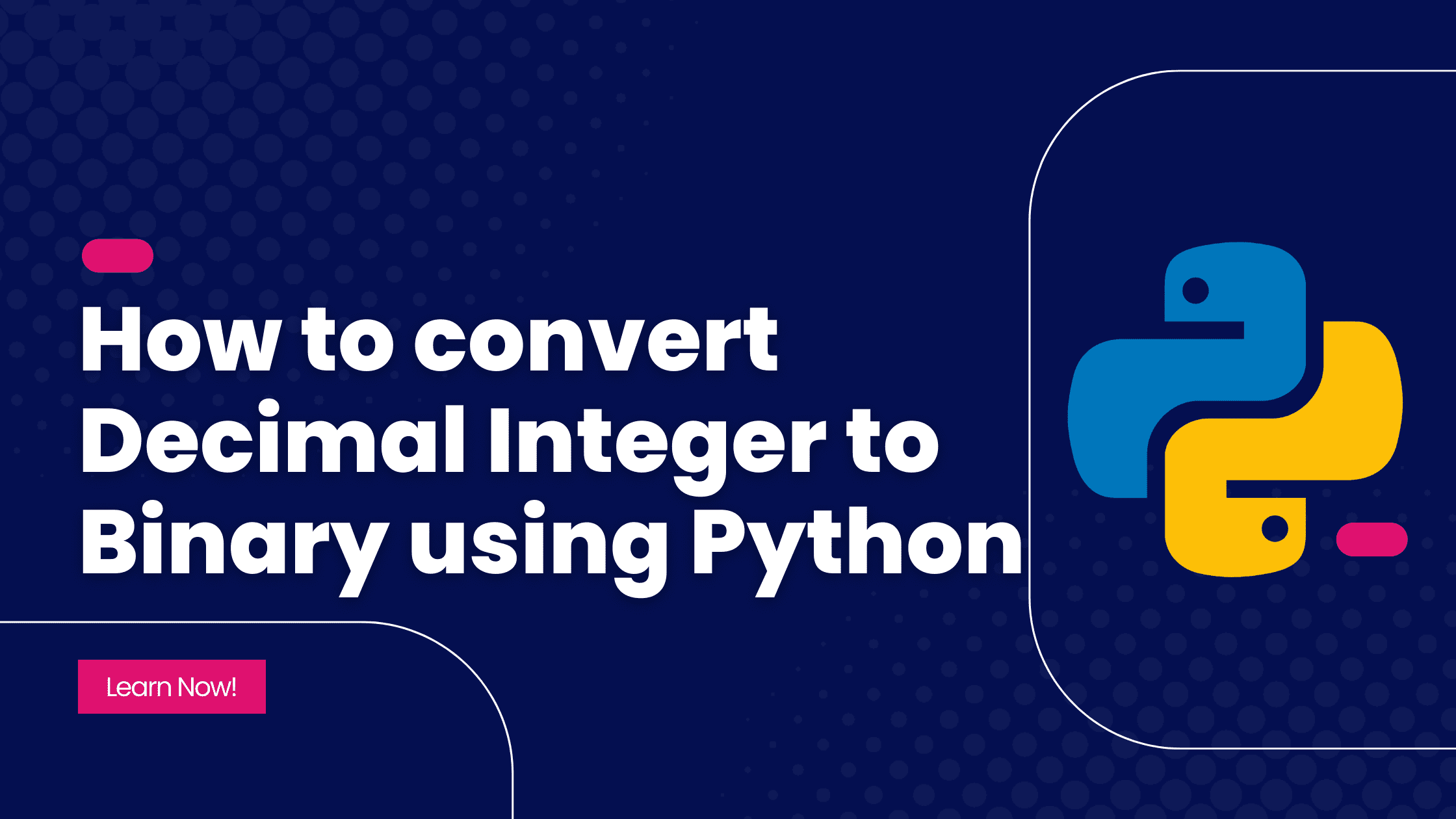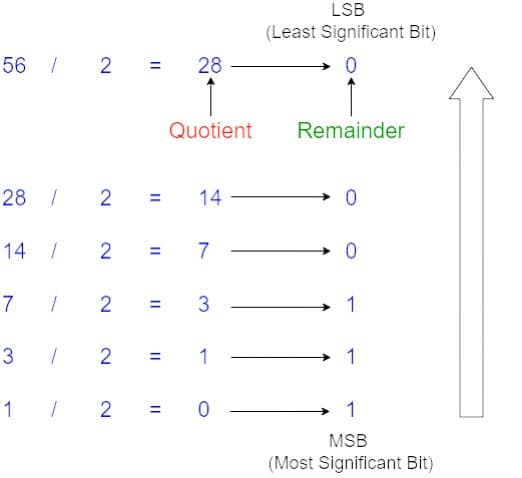# How to convert Decimal Integer to Binary in Python?

Data Structures PYTHON SHORTS SERIES# Introduction

We often need to convert decimal numbers into binary. For this purpose, Python has a built-in function called `bin`. But, in this tutorial, we will not be using this function. Instead, we will be using the Division by 2 method and the Stack data structure to convert decimal integers to their binary equivalent.

# What is the Division by 2 method?

Any decimal number when divided by 2 always leaves 0 or 1 as a remainder behind. The binary equivalent of the decimal number is therefore a string of 0s and 1s, which is produced by repeatedly dividing by 2.

Suppose, we are required to convert a decimal integer D into binary. When we divide D by 2, we will get a quotient D1 and a remainder r1 whose value can be either 0 or 1.

i.e. D = 2 * D1 + r1, where D1 is quotient and r1 is the remainder (0 or 1).

For example, we have a decimal number 56. Let's see how the method works.Now to get the binary equivalent, we need to read the remainders from Most Significant Bit to Least Significant Bit. Hence, for 56, we get 111000.

# Let's Code It!

Now, let us code our solution. To implement the division by 2 method, we will use the Stack data structure. If you're new to it, go through this tutorial first.

As we discussed above, we will keep calculating the remainder and pushing it to the stack repeatedly until the quotient becomes zero. Once the quotient becomes zero, we will start popping out the elements from the stack and append them to a binary string.

``````from stack import Stack

def convert_decimal_to_binary(decimal_integer):
stack = Stack()

while decimal_integer > 0:
remainder = decimal_integer % 2
stack.push(remainder)
decimal_integer = decimal_integer // 2

binary_number = ""
while not stack.is_empty():
binary_number += str(stack.pop())

return binary_number

if __name__ == "__main__":
print(convert_decimal_to_binary(10))
print(convert_decimal_to_binary(56))
print(int(convert_decimal_to_binary(256), 2) == 256)
``````

In the `convert_decimal_to_binary` function, we are first creating an empty stack. Now in lines 7-10, we get the remainder using the modulo operator and push it into the stack. Further, we divide the number by 2 to get the quotient and assign it to the same variable. Next, on line 12, we declare an empty string `binary_number`. Then we execute a while loop until the stack is empty. In the loop, we pop the topmost element and append it to the string `binary_number`. Once the stack is empty, the loop is terminated. The `binary_number` is returned on line 16.

In the main function, we test our implementation of the function.

# Conclusion

In this article, we learned how to convert decimal integers to binary without using any built-in function. We used the Stack data structure and the division by 2 method for this purpose. I hope, the tutorial was pretty clear to you.